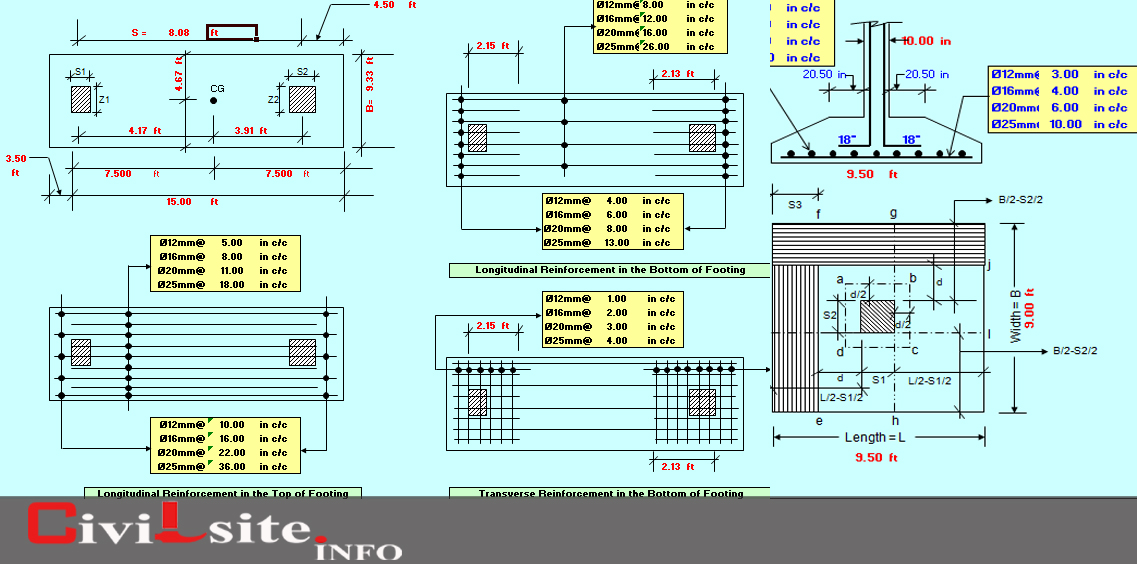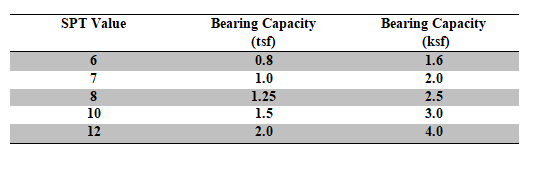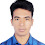# Footing Design Calculations - Shallow Foundation

Determination,
• Area of Footing (a)
• Depth of Footing (d)
• Short form of Footing Designs

## Footing Designs Calculation - Shallow Foundation:Footing Designs Calculation

#### Bearing Capacities of the Shallow Foundation from SPT:

The bearing capacities of the shallow foundation particularly for top layer of cohesive soil may be estimated from the SPT values, as suggested by terzaghi, according to following:Bearing capacities of the shallow foundation from SPTBearing capacities of the shallow foundation from SPT (Easiest form)Allowable bearing capacities of various foundation beds, (tsf)
Remark:Soils unable to carry 2000 psf generally requires pilling.

### Design procedure of Isometric Footing:

• Step 1: Determination of area of the Footing

Assuming, P = 225k ; qu = 2.5 ksf

So, the area of the footing = (Total Load)/(Bearing Capacity).

= (P * 1.1)/qu.

= (225* 1.1)/(2.5)

= 99 ft  ≈ 100 ft.

[Assuming, self-wt. of the footing 10%]

Total load = P+(10/100)*P   = P(1+0.1)

B = √100 = 10 ft.

So, Size of the footing is “10 ft X 10 ft”

Area of the Footing is 100 Sq Ft.

• Step 2: Determination of Depth of the footing

Punching Shear CheckArea of punching shear (Plan)Area of punching shear (Elevation)

Perimeter, P0(for square footing) = {(d +b) + (d +b)} * 2

= 2 (d +b) * 2

=4 (d +b)

P0 (for rectangular footing) = 2(b_1 +b_2) + 4d

So the resisting punching load = P0.d. 100⁄1000

= 4 (d +b) * d * 100⁄1000

= 4 (18 +15) * d * 100⁄1000

= 237.6k > 225k

Some loads can be deducted from the actual load to reduce the depth of the footing which brings economy by ensuring safety.

Net pressure = Lad/(Area of the footing)  = 225/(10 ft * 10 ft) = 2.25 ksf

Now, the modified pressure ={(102 + 2.752)} * 2.25 = 208 ksf

[Assuming, d = 18 inch; (18+15) = 33 inch = 2.75 ft]

Now,  P'/P = 0.92≈0.90

So, the load can be taken = 0.9 * P = 0.9 * 225 = 202.5 ≈ 205k

The short formula for determining the depth is

80<(Modified Load)/(P' * d)<90 = 80 <(205 * 1000)/( 4(b+d)  * d)<90

## Short form for designing the footing:

Determine width,        B = √((225 X 1.1)/(2.5)) ≈ 10 ft

And then trial,

For square footing,                   80 <(225 * 1000 * 0.9)/( 4(b+d)  * d)<90

For rectangular footing,         80 <(225 * 1000 * 0.9)/({2(b_1  + b_2 )+ 4d}* d)<90

#### Determination of Moments:

You also know pile cap design calculations details, Thank you.

1.1.2.1.3.